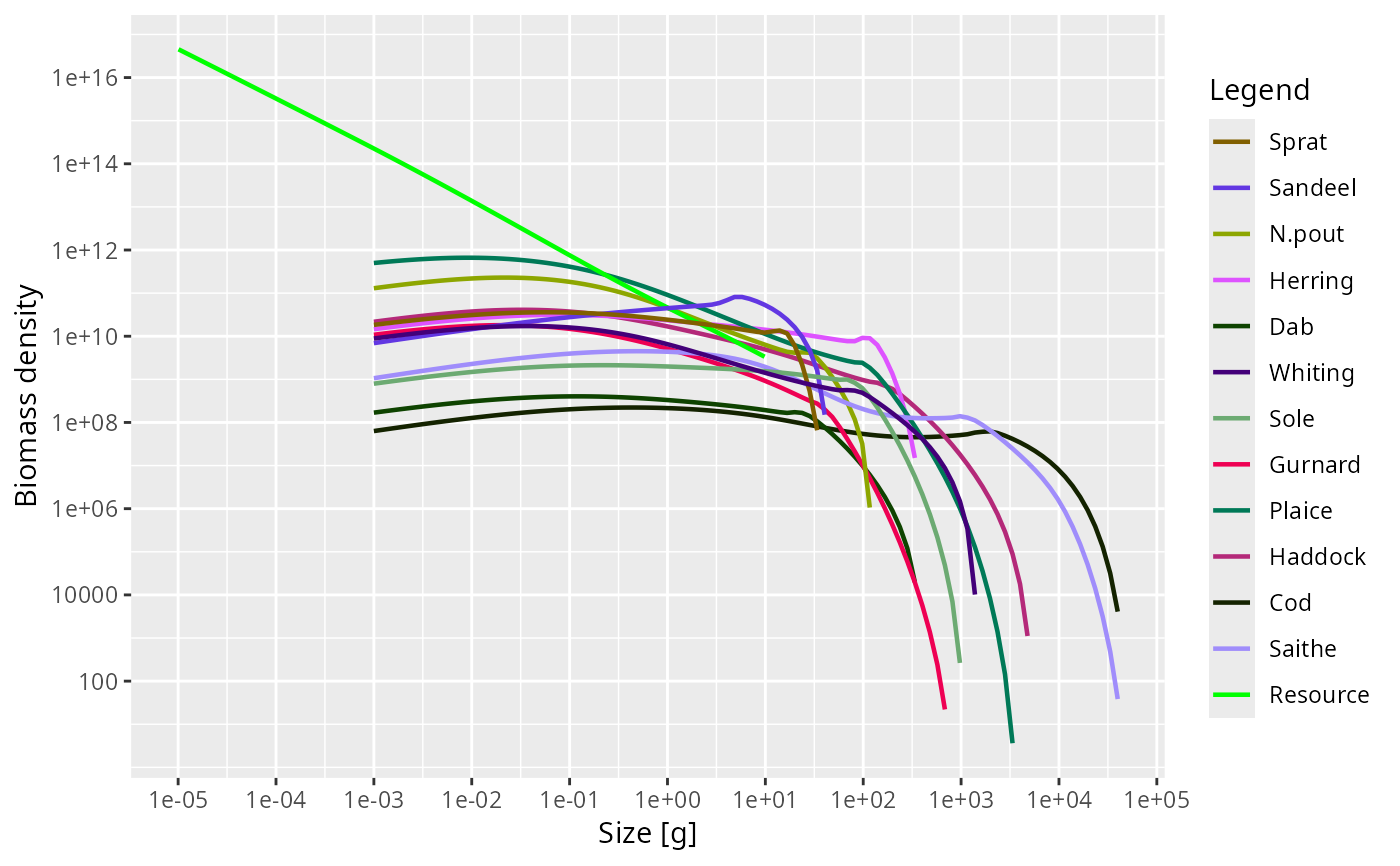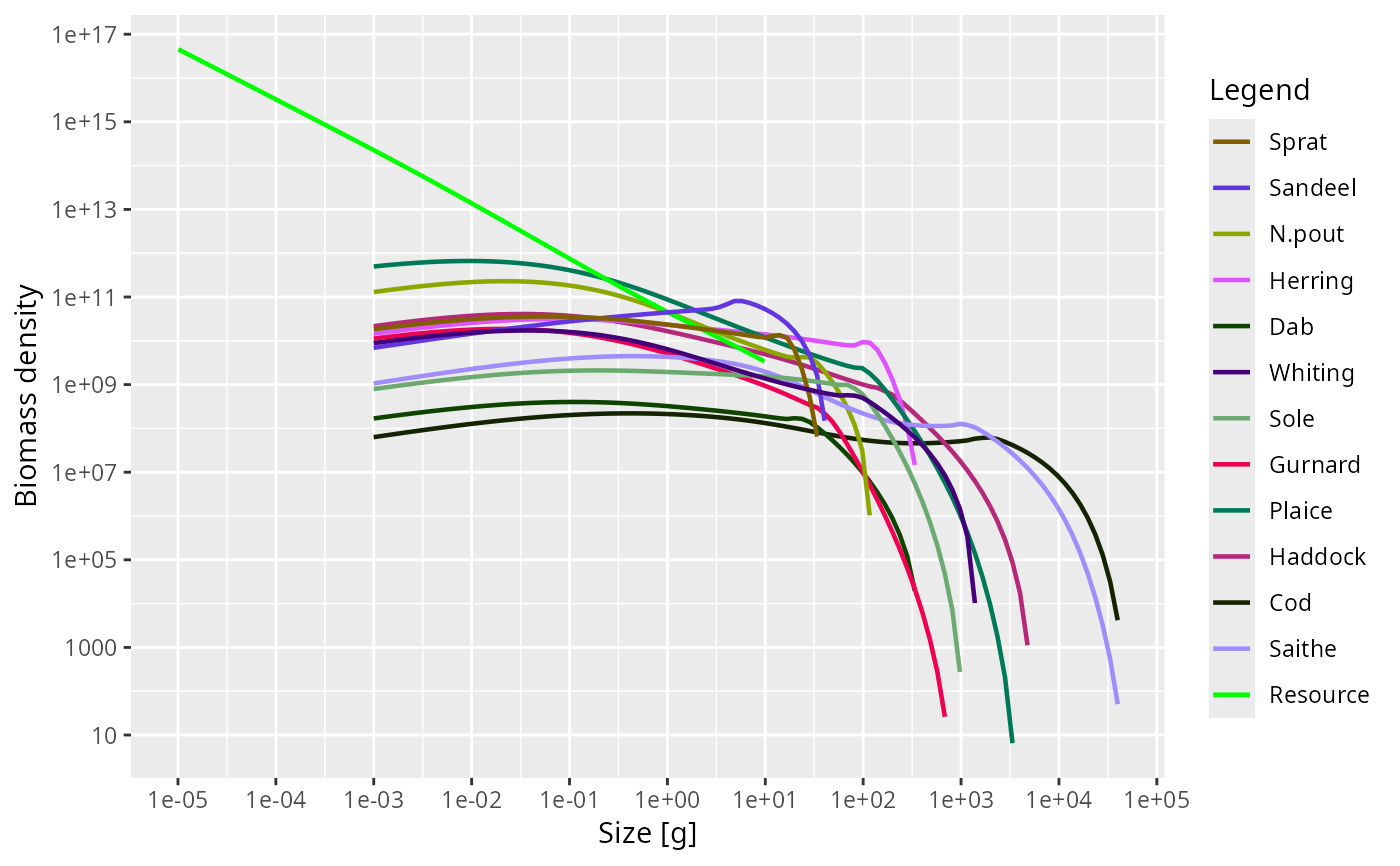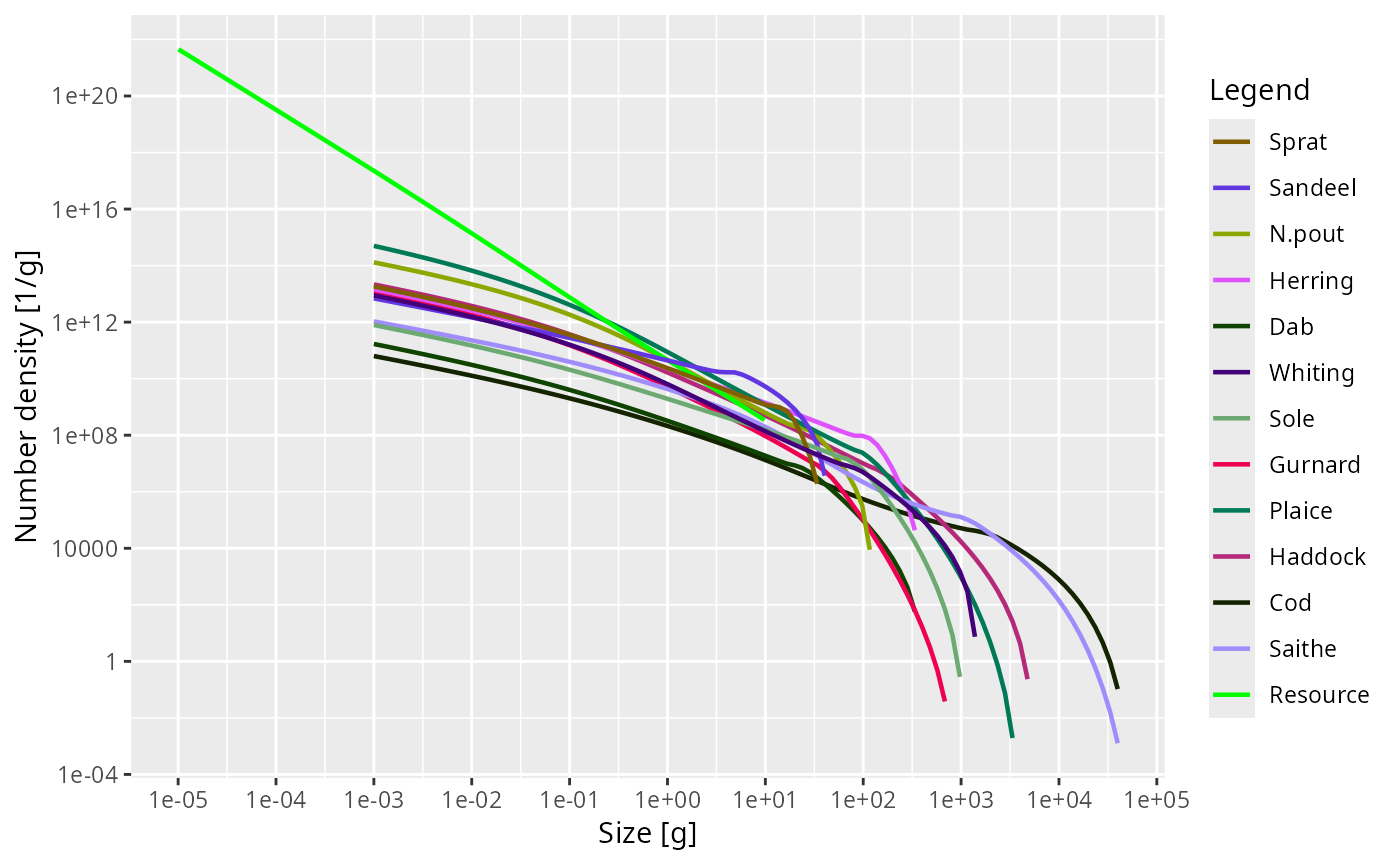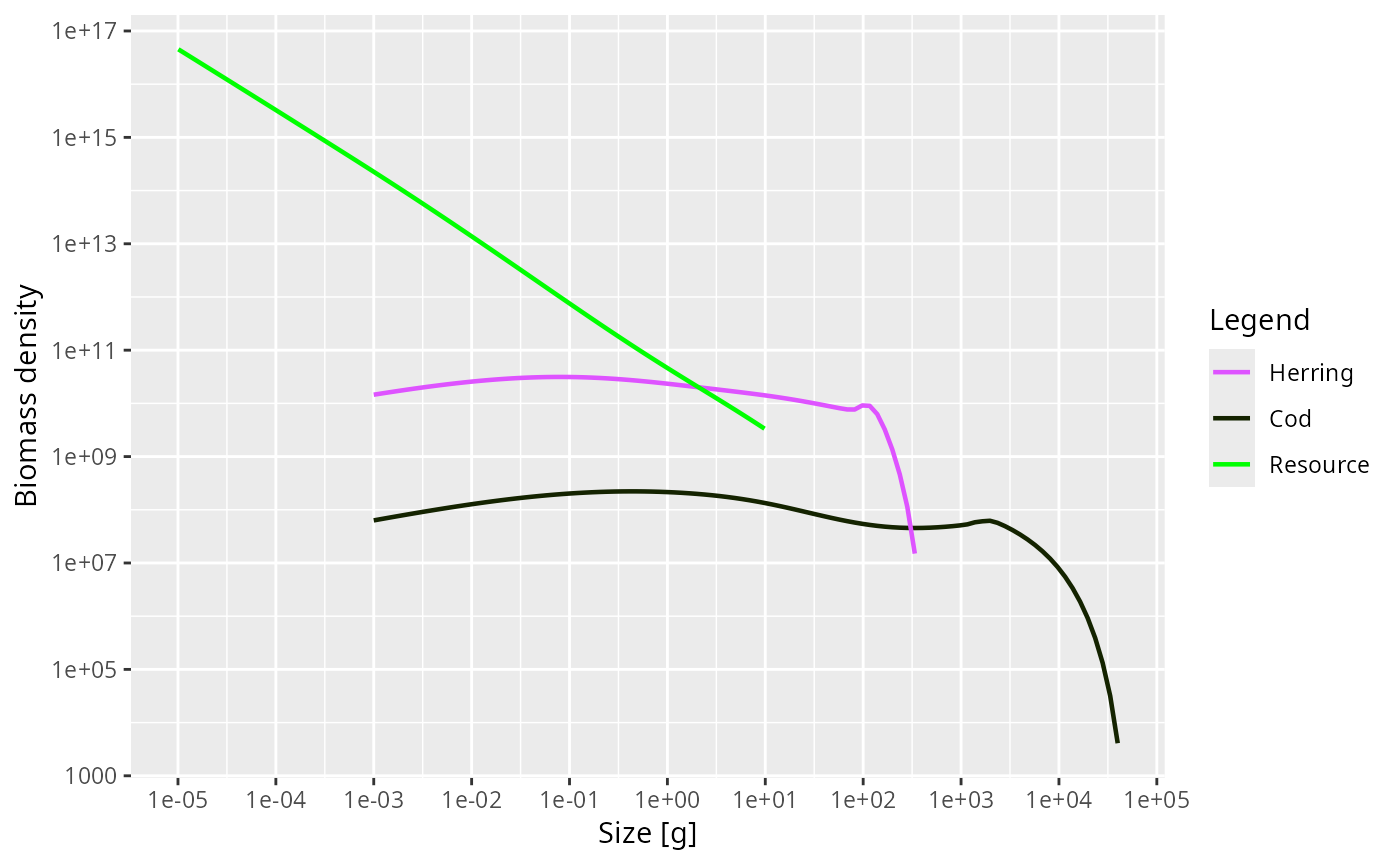Plots the number density multiplied by a power of the weight, with the power specified by the power argument.

plotSpectra(
object,
species = NULL,
time_range,
wlim = c(NA, NA),
ylim = c(NA, NA),
power = 1,
biomass = TRUE,
total = FALSE,
resource = TRUE,
background = TRUE,
highlight = NULL,
return_data = FALSE,
...
)

plotlySpectra(
object,
species = NULL,
time_range,
wlim = c(NA, NA),
ylim = c(NA, NA),
power = 1,
biomass = TRUE,
total = FALSE,
resource = TRUE,
background = TRUE,
highlight = NULL,
...
)

## Arguments

object An object of class MizerSim or MizerParams. The species to be selected. Optional. By default all target species are selected. A vector of species names, or a numeric vector with the species indices, or a logical vector indicating for each species whether it is to be selected (TRUE) or not. The time range (either a vector of values, a vector of min and max time, or a single value) to average the abundances over. Default is the final time step. Ignored when called with a MizerParams object. A numeric vector of length two providing lower and upper limits for the w axis. Use NA to refer to the existing minimum or maximum. A numeric vector of length two providing lower and upper limits for the y axis. Use NA to refer to the existing minimum or maximum. Any values below 1e-20 are always cut off. The abundance is plotted as the number density times the weight raised to power. The default power = 1 gives the biomass density, whereas power = 2 gives the biomass density with respect to logarithmic size bins.Only used if power argument is missing. Then biomass = TRUE is equivalent to power=1 and biomass = FALSE is equivalent to power=0 A boolean value that determines whether the total over all species in the system is plotted as well. Note that even if the plot only shows a selection of species, the total is including all species. Default is FALSE. A boolean value that determines whether resource is included. Default is TRUE. A boolean value that determines whether background species are included. Ignored if the model does not contain background species. Default is TRUE. Name or vector of names of the species to be highlighted. A boolean value that determines whether the formatted data used for the plot is returned instead of the plot itself. Default value is FALSE Other arguments (currently unused)

## Value

A ggplot2 object, unless return_data = TRUE, in which case a data frame with the four variables 'w', 'value', 'Species', 'Legend' is returned.

## Details

When called with a MizerSim object, the abundance is averaged over the specified time range (a single value for the time range can be used to plot a single time step). When called with a MizerParams object the initial abundance is plotted.

plotting_functions

Other plotting functions: animateSpectra(), plot,MizerSim,missing-method, plotBiomass(), plotDiet(), plotFMort(), plotFeedingLevel(), plotGrowthCurves(), plotPredMort(), plotYieldGear(), plotYield(), plotting_functions

## Examples

# \donttest{
params <-  NS_params
sim <- project(params, effort=1, t_max=20, t_save = 2, progress_bar = FALSE)
plotSpectra(sim)plotSpectra(sim, wlim = c(1e-6, NA))plotSpectra(sim, time_range = 10:20)plotSpectra(sim, time_range = 10:20, power = 0)plotSpectra(sim, species = c("Cod", "Herring"), power = 1)# Returning the data frame
fr <- plotSpectra(sim, return_data = TRUE)
str(fr)
#> 'data.frame':	1024 obs. of  4 variables:
#>  $w : num 0.001 0.001 0.001 0.001 0.001 0.001 0.001 0.001 0.001 0.001 ... #>$ value  : num  1.83e+10 6.92e+09 1.30e+11 1.46e+10 1.69e+08 ...
#>  $Species: chr "Sprat" "Sandeel" "N.pout" "Herring" ... #>$ Legend : chr  "Sprat" "Sandeel" "N.pout" "Herring" ...# }